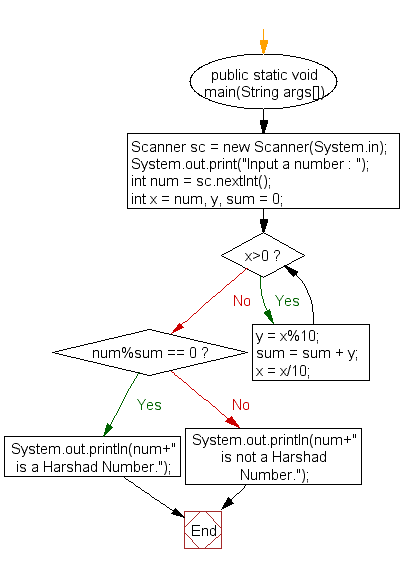﻿ Java exercises: Check whether a number is a Harshad Number or not - w3resource# Java Exercises: Check whether a number is a Harshad Number or not

## Java Numbers: Exercise-12 with Solution

Write a Java program to check whether a number is a Harshad Number or not.

In recreational mathematics, a harshad number in a given number base, is an integer that is divisible by the sum of its digits when written in that base.
Example: Number 200 is a Harshad Number because the sum of digits 2 and 0 and 0 is 2(2+0+0) and 200 is divisible by 2. Number 171 is a Harshad Number because the sum of digits 1 and 7 and 1 is 9(1+7+1) and 171 is divisible by 9.

Test Data
Input a number : 353

Pictorial Presentation:Sample Solution:

Java Code:

``````import java.util.Scanner;
public class Example12 {

public static void main(String args[])
{
Scanner sc = new Scanner(System.in);

System.out.print("Input a number : ");
int num = sc.nextInt();
int x = num, y, sum = 0;

while(x>0)
{
y = x%10;
sum = sum + y;
x = x/10;
}

if(num%sum == 0)
else
System.out.println(num+" is not a Harshad Number.");
}
}
```
```

Sample Output:

```Input a number : 353
353 is not a Harshad Number.
```

Flowchart:Java Code Editor:

What is the difficulty level of this exercise?

﻿

## Java: Tips of the Day

Array vs ArrayLists:

The main difference between these two is that an Array is of fixed size so once you have created an Array you cannot change it but the ArrayList is not of fixed size. You can create instances of ArrayLists without specifying its size. So if you create such instances of an ArrayList without specifying its size Java will create an instance of an ArrayList of default size.

Once an ArrayList is full it re-sizes itself. In fact, an ArrayList is internally supported by an array. So when an ArrayList is resized it will slow down its performance a bit as the contents of the old Array must be copied to a new Array.

At the same time, it's compulsory to specify the size of an Array directly or indirectly while creating it. And also Arrays can store both primitives and objects while ArrayLists only can store objects.

Ref: https://bit.ly/3o8L2KH